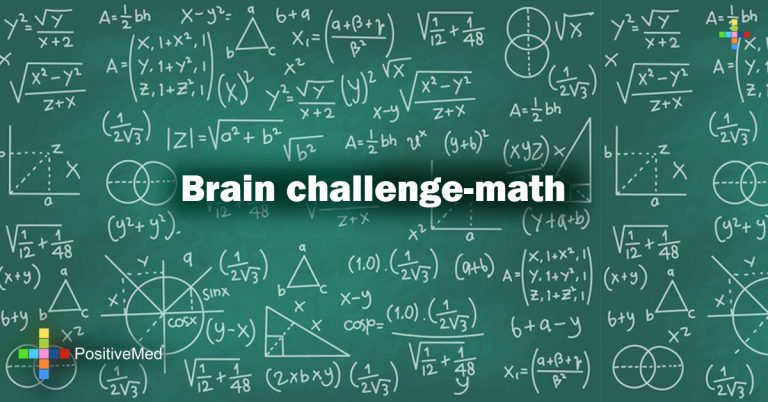Home games Brain challenge-math

### Brain challenge-mathComplete the equations using the numbers 1-16. Each number can only be used once.
Each row is a math equation that results in the given number at the end of the row.
Each column is also a math equation that its answer is shown at the end of the column.Remember, multiplication and division are always done before addition and subtraction, no matter where are they are located in the problem.